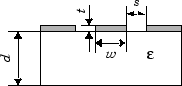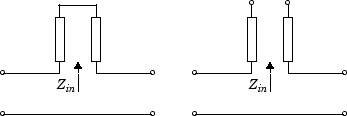# rfckt.cpw

Coplanar waveguide transmission line

## Description

Use the `cpw` object to represent coplanar waveguide transmission lines that are characterized by line dimensions, stub type, and termination.

A coplanar waveguide transmission line is shown in cross-section in the following figure. Its physical characteristics include the conductor width (w), the conductor thickness (t), the slot width (s), the substrate height (d), and the permittivity constant (ε).## Creation

### Syntax

``h = rfckt.cpw``
``h = rfckt.cpw(Name,Value)``

### Description

example

````h = rfckt.cpw` returns a coplanar waveguide transmission line object whose properties are set to their default values.```
````h = rfckt.cpw(Name,Value)` sets properties using one or more name-value pairs. For example, `rfckt.cpw('ConductorWidth',0.3)` creates an RF coplanar waveguide transmission line with a width of 0.3 meters. You can specify multiple name-value pairs. Enclose each property name in a quote. Properties not specified retain their default values.```

## Properties

expand all

Computed S-parameters, noise figure, OIP3, and group delay values, specified as an `rfdata.data` object. For more information refer, Algorithms.

Data Types: `function_handle`

Physical width of conductor, specified as a scalar in meters. By default, the value is `0.6e-4`.

Data Types: `double`

Relative permittivity of dielectric, specified as a scalar. The relative permittivity is the ratio of permittivity of the dielectric,$\epsilon$, to the permittivity in free space, ${\epsilon }_{0}$. By default, the value is `9.8`.

Data Types: `double`

Dielectric thickness or physical height of the conductor, specified as a scalar in meters. The default value is `0.635e-4`.

Data Types: `double`

Physical length of transmission, specified as a scalar in meters. The default value is `0.01`.

Data Types: `double`

Loss angle tangent of dielectric, specified as a scalar. The default value is `0`.

Data Types: `double`

Name of coplanar waveguide transmission line object, specified as a `1-by-N` character array.

Data Types: `char`

Number of ports, specified as a positive integer.

Data Types: `double`

Conductor conductivity, specified as a scalar in Siemens per meter (S/m). The default value is `Inf`.

Data Types: `double`

Type of stub, specified as one of the following values: `'NotaStub'`, `'Series'`, `'Shunt'`.

Data Types: `double`

Physical width of slot, specified as a scalar in meters. The default value is `0.2e-4`.

Data Types: `double`

Stub transmission line termination, specified as one of the following values: `'NotApplicable'`, `'Open'`, `'Short'`.

Data Types: `double`

Physical thickness of conductor, specified as a scalar in meters. The default value is `0.005e-6`.

Data Types: `double`

## Object Functions

 `analyze` Analyze RFCKT object in frequency domain `calculate` Calculate specified parameters for rfckt objects or rfdata objects `circle` Draw circles on Smith Chart `extract` Extract specified network parameters from rfckt object or data object `listformat` List valid formats for specified circuit object parameter `listparam` List valid parameters for specified circuit object `loglog` Plot specified circuit object parameters using log-log scale `plot` Plot specified circuit object parameters on X-Y plane `plotyy` Plot parameters of RF circuit or RF data on X-Y plane with Y-axes on both left and right sides `getop` Display operating conditions `polar` Plot specified object parameters on polar coordinates `semilogx` Plot RF circuit object parameters using log scale for x-axis `semilogy` Plot RF circuit object parameters using log scale for y-axis `smith` Plot circuit object parameters on Smith chart `write` Write RF data from circuit or data object to file `getz0` Get characteristic impedance of transmission line object `read` Read RF data from file to new or existing circuit or data object `restore` Restore data to original frequencies `getop` Display operating conditions

## Examples

collapse all

Create a coplanar waveguide transmission line using rfckt.cpw.

`tx=rfckt.cpw('Thickness',0.0075e-6)`
```tx = rfckt.cpw with properties: ConductorWidth: 6.0000e-04 SlotWidth: 2.0000e-04 Height: 6.3500e-04 Thickness: 7.5000e-09 EpsilonR: 9.8000 LossTangent: 0 SigmaCond: Inf LineLength: 0.0100 StubMode: 'NotAStub' Termination: 'NotApplicable' nPort: 2 AnalyzedResult: [] Name: 'Coplanar Waveguide Transmission Line' ```

## Algorithms

The `analyze` method treats the transmission line as a 2-port linear network. It computes the `AnalyzedResult` property of a stub or as a stub less line using the data stored in the `rfckt.cpw` object properties as follows:

• If you model the transmission line as a stub less line, the `analyze` method first calculates the ABCD-parameters at each frequency contained in the modeling frequencies vector. It then uses the `abcd2s` function to convert the ABCD-parameters to S-parameters.

The `analyze` method calculates the ABCD-parameters using the physical length of the transmission line, d, and the complex propagation constant, k, using the following equations:

`$\begin{array}{l}A=\frac{{e}^{kd}+{e}^{-kd}}{2}\\ B=\frac{{Z}_{0}*\left({e}^{kd}-{e}^{-kd}\right)}{2}\\ C=\frac{{e}^{kd}-{e}^{-kd}}{2*{Z}_{0}}\\ D=\frac{{e}^{kd}+{e}^{-kd}}{2}\end{array}$`

Z0 and k are vectors whose elements correspond to the elements of f, the vector of frequencies specified in the `analyze` input argument `freq`. Both can be expressed in terms of the specified conductor strip width, slot width, substrate height, conductor strip thickness, relative permittivity constant, conductivity and dielectric loss tangent of the transmission line, as described in .

• If you model the transmission line as a shunt or series stub, the `analyze` method first calculates the ABCD-parameters at the specified frequencies. It then uses the `abcd2s` function to convert the ABCD-parameters to S-parameters.

When you set the `StubMode` property to `'Shunt'`, the 2-port network consists of a stub transmission line that you can terminate with either a short circuit or an open circuit as shown in the following figure.Zin is the input impedance of the shunt circuit. The ABCD-parameters for the shunt stub are calculated as:

`$\begin{array}{c}A=1\\ B=0\\ C=1/{Z}_{in}\\ D=1\end{array}$`

When you set the `StubMode` property to `'Series'`, the 2-port network consists of a series transmission line that you can terminate with either a short circuit or an open circuit as shown in the following figure.Zin is the input impedance of the series circuit. The ABCD-parameters for the series stub are calculated as:

`$\begin{array}{c}A=1\\ B={Z}_{in}\\ C=0\\ D=1\end{array}$`

The `analyze` method uses the S-parameters to calculate the group delay values at the frequencies specified in the `analyze` input argument `freq`, as described in the `analyze` reference page.

  Gupta, K. C., R. Garg, I. Bahl, and P. Bhartia, Microstrip Lines and Slotlines, 2nd Edition, Artech House, Inc., Norwood, MA, 1996.